GFG App
Open AppBrowser
Continue

In this article, we will implement the 2:4 Decoder using all levels of abstraction in Verilog HDL with a step-by-step procedure. Before proceeding to code we shall look into the truth table and logic symbol of the 2:4 Decoder.

### 2:4 Decoder

A decoder is a combinational logic circuit that has ‘n’ input signal lines and 2n output lines. In the 2:4 decoder, we have 2 input lines and 4 output lines. In addition, we provide ‘enable‘ to the input to ensure the decoder is functioning whenever enable is 1 and it is turned off when enable is 0. The truth table, logic diagram, and logic symbol are given below:

#### Logic Symbol: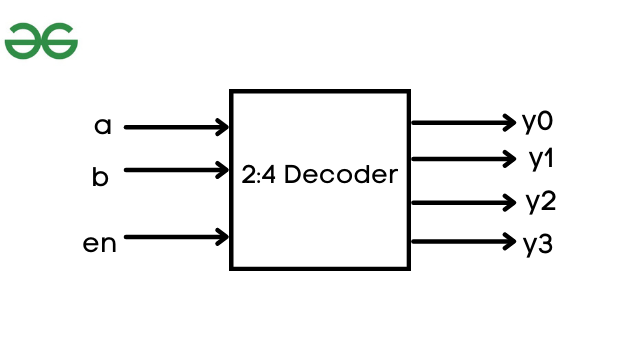#### Logic Diagram: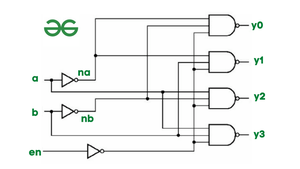Similar to the 2:4 decoder, 3 to 8 decoder produces eight output signal lines, and 4 to 16 decoder produces sixteen output signal lines.

We shall now implement a 2:4 decoder in different levels of abstraction from highest to lowest.

### 1. Behavioral Modeling:

Behavioral modeling represents the circuit at a high level of abstraction. The syntax of Behavioral modeling resembles that of C programming. We can implement conditions statements like if, case statement, looping, and structure procedures(initial and always) within the Verilog design block using Behavioral modeling.

#### Syntax:

```if(condition)
true_statement;
else if(condition)
true_statement;
else
else_statement```

#### Design Block: Behavioral Modeling

```module decoder24_behaviour(en,a,b,y);
// input port
input en,a,b;

// use reg to store the output value
output reg [3:0]y;
// always is used in design block
// only in Behavioural modeling.

always @(en,a,b)
begin
// using condition if statement
// implement the 2:4 truth table
if(en==0)
begin
if(a==1'b0 & b==1'b0) y=4'b1110;
else if(a==1'b0 & b==1'b1) y=4'b1101;
else if(a==1'b1 & b==1'b0) y=4'b1011;
else if(a==1 & b==1) y=4'b0111;
else y=4'bxxxx;
end
else
y=4'b1111;
end
endmodule```

### Testbench: Behavioral Modeling

Once we have designed the design block we must test it. The testbench is a simulation block that is used to test the design block by applying stimulus and checking results bypassing input values. Think Design block as the output implementation where we only think of the output result without worrying about input. But while writing testbench you need to give proper inputs, here the truth table is very important to declare input values.

In Testbench the important step is to link the design block, this is done by instantiation:

`modulename instance(port list);`

To display the result we shall use the \$monitor system task.

#### Syntax:

`\$monitor(display_statement);`
```module tb;

// input port are declared in reg(register)
reg a,b,en;

// output port are declared in wire(net)
wire [3:0]y;

// instantiate design block
decoder24_behaviour dut(en,a,b,y);

initial
begin
\$monitor("en=%b a=%b b=%b y=%b",en,a,b,y);
// with reference to truth table provide input values
en=1;a=1'bx;b=1'bx;#5
en=0;a=0;b=0;#5
en=0;a=0;b=1;#5
en=0;a=1;b=0;#5
en=0;a=1;b=1;#5

// terminate simulation using \$finish system task
\$finish;
end
endmodule```

Output: To run the program:

```iverilog -o behavior tb.v dut.v
vvp behavior```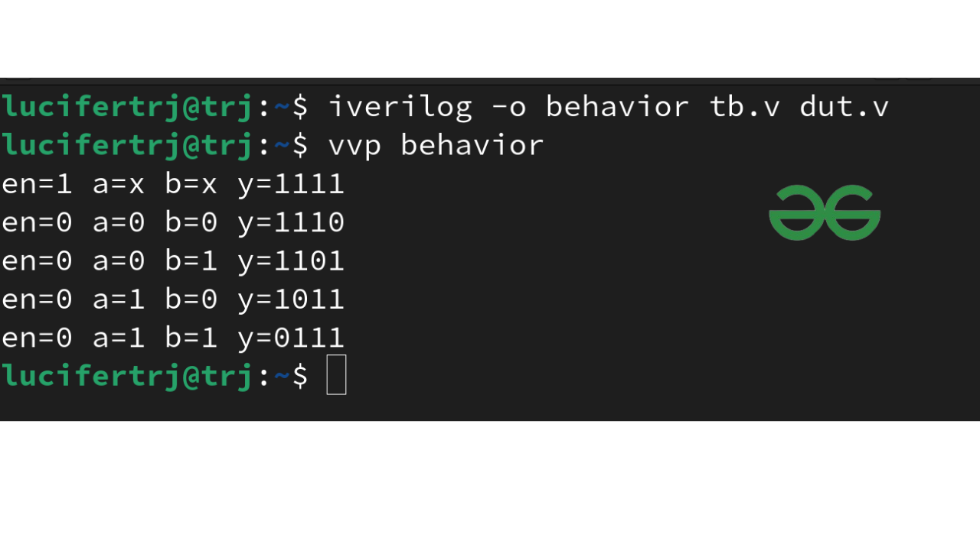Note: iverilog is the Verilog compiler to run Verilog programs. vvp is the command to run the Verilog code.

### 2. Data Flow Modeling:

In defining Data Flow Modeling a designer has to endure in mind how data flows within the design description. Dataflow modeling has become a well-liked design approach, as logic synthesis tools became refined. In Data Flow we use keyword assign to store the net values.

#### Syntax:

`assign out = expression;`

#### Design Block: Data Flow

```module decoder24_assign(en,a,b,y);
// declare input and output ports
input en,a,b;
output [3:0]y;

// supportive connection required
wire enb,na,nb;
assign enb = ~en;
assign na = ~a;
assign nb = ~b;

// assign output value by referring to logic diagram
assign y = ~(enb&na&nb);
assign y = ~(enb&na&b);
assign y = ~(enb&a&nb);
assign y = ~(enb&a&b);

endmodule```

#### TestBench: Data Flow

```module tb;
// input port are declared in reg(register)
reg a,b,en;

// output port are declared in wire(net)
wire [3:0]y;

// instantiate design block
decoder24_assign dut(en,a,b,y);

initial
begin
\$monitor("en=%b a=%b b=%b y=%b",en,a,b,y);
// with reference to truth
// table provide input values
en=1;a=1'bx;b=1'bx;#5
en=0;a=0;b=0;#5
en=0;a=0;b=1;#5
en=0;a=1;b=0;#5
en=0;a=1;b=1;#5
// terminate simulation using \$finish system task
\$finish;
end
endmodule```

Output: To Run the Program:

```iverilog -o assign tb.v dut.v
vvp assign```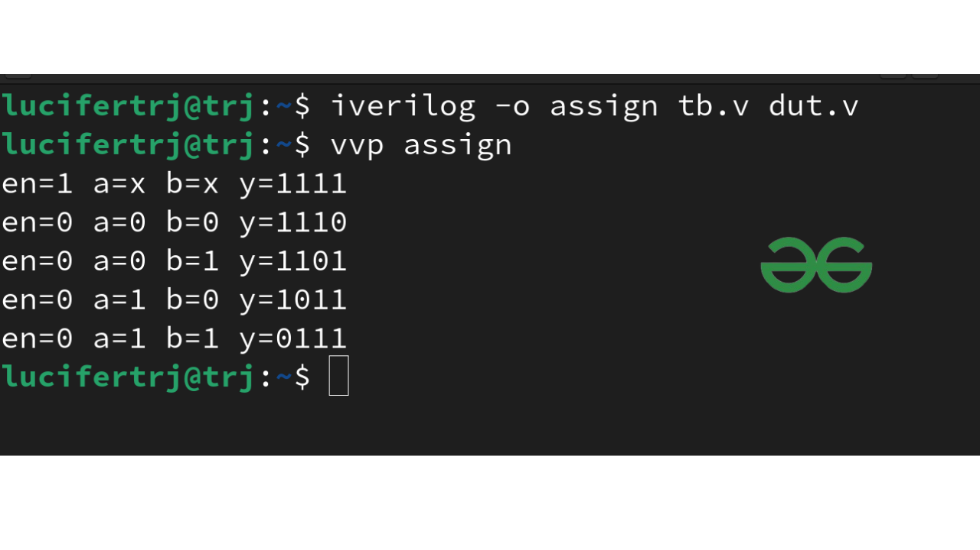### 3. Gate Level Modeling:

Logic gates are building blocks of logic circuits. Verilog supports basic gates as predefined primitives. These primitives are instantiated(creating instance/object) and can be implemented within the module definition.

#### Syntax:

`logicgate object(output_port,input_port)`

#### Example:

`nand n1(y,x1,x2)`

Note: Logic gates are in lower case and the object can be any name other than keywords. In the above example, y is output, and x1 and x2 are input signals.

Verilog code starts with module definition with input and output ports passed as the argument. With help of the logic diagram, we shall instantiate 4 NAND gates and 3 NOT gate to connect input and output signals to implement the 2:4 Decoder.

#### Design Block: Gate Level

```module decoder24_gate(en,a,b,y);
// declare input and output ports
input en,a,b;
output [3:0]y;

// supportive connections required
// to connect nand gates
wire enb,na,nb;

// instantiate 4 nand gates and 3 not gates
// make connections by referring the above logic diagram
not n0(enb,en);
not n1(na,a);
not n2(nb,b);

nand n3(y,enb,na,nb);
nand n4(y,enb,na,b);
nand n5(y,enb,a,nb);
nand n6(y,enb,a,b);

endmodule```

#### Testbench: Gate Level

```module tb;
// input port are declared in reg(register)
reg a,b,en;
// output port are declared in wire(net)
wire [3:0]y;
// instantiate design block
decoder24_gate dut(en,a,b,y);

initial
begin
\$monitor("en=%b a=%b b=%b y=%b",en,a,b,y);
// with reference to truth table provide input values
en=1;a=1'bx;b=1'bx;#5
en=0;a=0;b=0;#5
en=0;a=0;b=1;#5
en=0;a=1;b=0;#5
en=0;a=1;b=1;#5
// terminate simulation using \$finish system task
\$finish;
end
endmodule```

Output: To run the program:

```iverilog -o gate tb.v dut.v
vvp gate```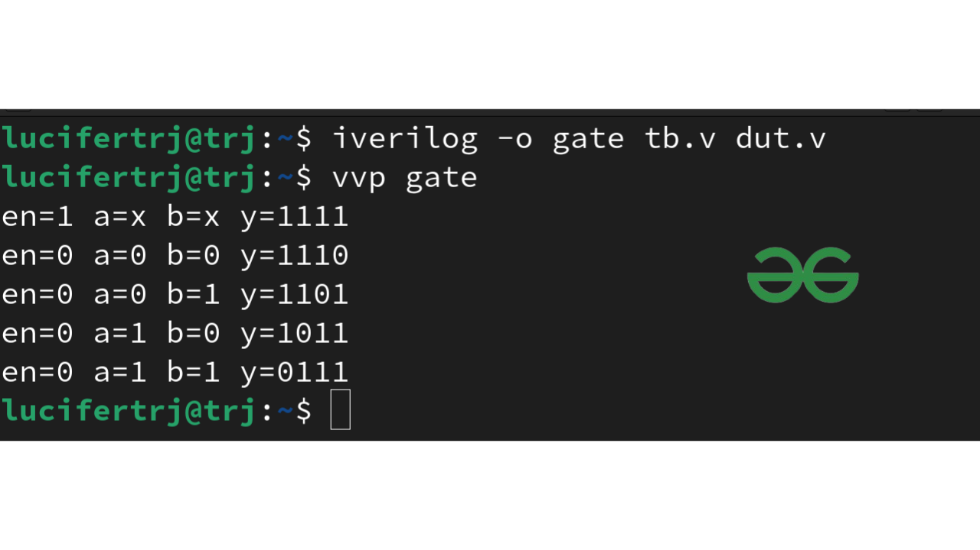### Application Of Decoder:

Here are some fields where the decoder is implemented in the real world:

1. Code Converters
2. Robotic Vehicle with Metal Detector
3. RF-based Home Automation System
4. Used in ALU of CPU
5. Speed Synchronization of Multiple Motors in Industries

My Personal Notes arrow_drop_up